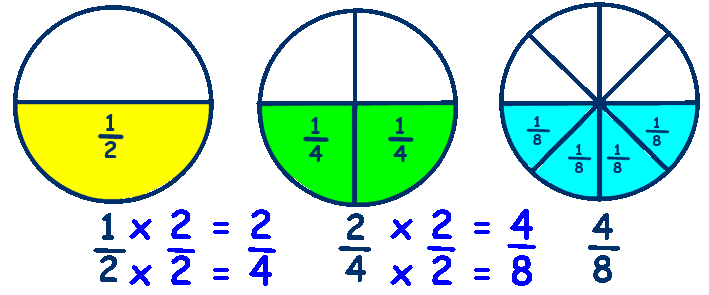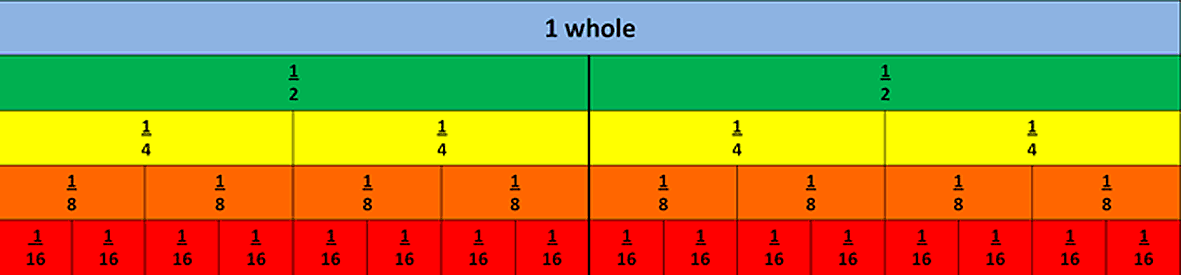# Equivalent Fractions

Look at these three fractions, 1/2, 2/4 and 4/8These three fractions all look different when written but all have the same value.

## They are Equivalent Fractions

If you multiply or divide the numerator and the denominator by the same number,
then the fraction remains the same and is said to be equivalent.A useful worksheet to practice with Equivalent Fractions and cut out and match up, Fractions Match Up plus an excellent Powerpoint presentation on Equivalent Fractions, from Primary Resources.

An alternative way of looking at Equivalent Fractions is by the use of a Fraction Wall.A Fraction Wall enables children to visualise how different fractions relate
to one another and Equivalent Fractions can be easily identified.

This excellent functional

Online Number Wall

is available from 'Maths is Fun', it demonstrates equivalent fractions down to sixteenths and is well worth a look.

A very useful exercise is to ask your child to create their own fraction wall - include fifths, sixths, tenths, twelfths etc.

____________________________________

Practice Equivalent Fractions with Dick and Dom from BBC Bitesize